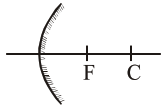# The focal length f`
Question:

The focal length $f$ is related to the radius of curvature $r$ of the spherical convex mirror by:

1. $f=+\frac{1}{2} r$

2. $f=-r$

3. $\mathrm{f}=-\frac{1}{2} \mathrm{r}$

4. $f=r$

Correct Option: 1

Solution:

For convex mirror, focus is behind the mirror.